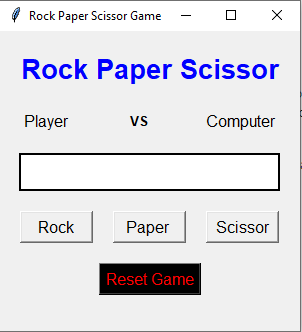# Rock Paper and Scissor Game Using Tkinter

• Last Updated : 13 Sep, 2021

Prerequisite: Tkinter, random

Python offers multiple options for developing a GUI (Graphical User Interface). Out of all the GUI methods, Tkinter is the most commonly used method. It is a standard Python interface to the Tk GUI toolkit shipped with Python. Python with Tkinter is the fastest and easiest way to create GUI applications.

In this article, we will see how we can create a rock paper and scissor game using Tkinter. Rock paper scissor is a hand game usually played between two people, in which each player simultaneously forms one of three shapes with an outstretched hand. These shapes are “rock”, “paper”, and “scissors”.

Game Winner Condition:

• Paper and Scissor =>Scissor win
• Rock and Scissor =>Rock win
• Paper and Rock =>Paper win

Below is what the GUI looks like:-GUI Implementation Steps (Add Button, Label, Frame)

• Create a head label that will show the title of the game, set its font and properties
• Below the head, label create a user label that will show the hand sign selected by the user
• Create a computer label that will show hand sign picked by the computer
• In between the user and the computer label create a label to show the text “vs”
• Create a result label to show the result set font and other properties to it
• Create three pushbuttons for rock, paper, and scissor respectively
• Create a reset button to reset the game

Backend Implementation Steps:

• We will create five functions; one is for reset the game, the second is for disabling the button and the other are for game winners

Below is the Implementation:-

## Python3

 `# Import Required Library``from` `tkinter ``import` `*``import` `random` `# Create Object``root ``=` `Tk()` `# Set geometry``root.geometry(``"300x300"``)` `# Set title``root.title(``"Rock Paper Scissor Game"``)` `# Computer Value``computer_value ``=` `{``    ``"0"``:``"Rock"``,``    ``"1"``:``"Paper"``,``    ``"2"``:``"Scissor"``}` `# Reset The Game``def` `reset_game():``    ``b1[``"state"``] ``=` `"active"``    ``b2[``"state"``] ``=` `"active"``    ``b3[``"state"``] ``=` `"active"``    ``l1.config(text ``=` `"Player              "``)``    ``l3.config(text ``=` `"Computer"``)``    ``l4.config(text ``=` `"")` `# Disable the Button``def` `button_disable():``    ``b1[``"state"``] ``=` `"disable"``    ``b2[``"state"``] ``=` `"disable"``    ``b3[``"state"``] ``=` `"disable"` `# If player selected rock``def` `isrock():``    ``c_v ``=` `computer_value[``str``(random.randint(``0``,``2``))]``    ``if` `c_v ``=``=` `"Rock"``:``        ``match_result ``=` `"Match Draw"``    ``elif` `c_v``=``=``"Scissor"``:``        ``match_result ``=` `"Player Win"``    ``else``:``        ``match_result ``=` `"Computer Win"``    ``l4.config(text ``=` `match_result)``    ``l1.config(text ``=` `"Rock            "``)``    ``l3.config(text ``=` `c_v)``    ``button_disable()` `# If player selected paper``def` `ispaper():``    ``c_v ``=` `computer_value[``str``(random.randint(``0``, ``2``))]``    ``if` `c_v ``=``=` `"Paper"``:``        ``match_result ``=` `"Match Draw"``    ``elif` `c_v``=``=``"Scissor"``:``        ``match_result ``=` `"Computer Win"``    ``else``:``        ``match_result ``=` `"Player Win"``    ``l4.config(text ``=` `match_result)``    ``l1.config(text ``=` `"Paper           "``)``    ``l3.config(text ``=` `c_v)``    ``button_disable()` `# If player selected scissor``def` `isscissor():``    ``c_v ``=` `computer_value[``str``(random.randint(``0``,``2``))]``    ``if` `c_v ``=``=` `"Rock"``:``        ``match_result ``=` `"Computer Win"``    ``elif` `c_v ``=``=` `"Scissor"``:``        ``match_result ``=` `"Match Draw"``    ``else``:``        ``match_result ``=` `"Player Win"``    ``l4.config(text ``=` `match_result)``    ``l1.config(text ``=` `"Scissor         "``)``    ``l3.config(text ``=` `c_v)``    ``button_disable()` `# Add Labels, Frames and Button``Label(root,``      ``text ``=` `"Rock Paper Scissor"``,``      ``font ``=` `"normal 20 bold"``,``      ``fg ``=` `"blue"``).pack(pady ``=` `20``)` `frame ``=` `Frame(root)``frame.pack()` `l1 ``=` `Label(frame,``           ``text ``=` `"Player              "``,``           ``font ``=` `10``)` `l2 ``=` `Label(frame,``           ``text ``=` `"VS             "``,``           ``font ``=` `"normal 10 bold"``)` `l3 ``=` `Label(frame, text ``=` `"Computer"``, font ``=` `10``)` `l1.pack(side ``=` `LEFT)``l2.pack(side ``=` `LEFT)``l3.pack()` `l4 ``=` `Label(root,``           ``text ``=` `"",``           ``font ``=` `"normal 20 bold"``,``           ``bg ``=` `"white"``,``           ``width ``=` `15` `,``           ``borderwidth ``=` `2``,``           ``relief ``=` `"solid"``)``l4.pack(pady ``=` `20``)` `frame1 ``=` `Frame(root)``frame1.pack()` `b1 ``=` `Button(frame1, text ``=` `"Rock"``,``            ``font ``=` `10``, width ``=` `7``,``            ``command ``=` `isrock)` `b2 ``=` `Button(frame1, text ``=` `"Paper "``,``            ``font ``=` `10``, width ``=` `7``,``            ``command ``=` `ispaper)` `b3 ``=` `Button(frame1, text ``=` `"Scissor"``,``            ``font ``=` `10``, width ``=` `7``,``            ``command ``=` `isscissor)` `b1.pack(side ``=` `LEFT, padx ``=` `10``)``b2.pack(side ``=` `LEFT,padx ``=` `10``)``b3.pack(padx ``=` `10``)` `Button(root, text ``=` `"Reset Game"``,``       ``font ``=` `10``, fg ``=` `"red"``,``       ``bg ``=` `"black"``, command ``=` `reset_game).pack(pady ``=` `20``)` `# Execute Tkinter``root.mainloop()`

Output:

My Personal Notes arrow_drop_up